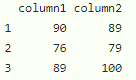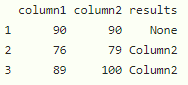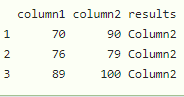Open in App
Not now

# How to Compare Two Columns in R DataFrame?

• Last Updated : 19 Dec, 2021

In this article, we will discuss how to compare two columns in the dataframe through R Programming Language.

We can compare two columns in R by using ifelse(). This statement is used to check the condition given and return the data accordingly.

Syntax:

`ifelse(df\$column1 > df\$column2, statement,............)`

where,

• df is the input dataframe
• column are the columns in the given dataframe

Example:

Let’s create the dataframe with two columns

## R

 `# dataframe ` `data = ``data.frame``(column1=``c``(90, 76, 89),  ` `                  ``column2=``c``(89, 79, 100)) ` ` `  `# display ` `data `

Output:Example 1:

Here , we are going to check if column1 value is greater and if greater then add a new column named results and assign with Column1. If column2 value is greater then add a new column named results and assign it with Column2. otherwise None

## R

 `# dataframe ` `data = ``data.frame``(column1=``c``(90, 76, 89), ` `                  ``column2=``c``(90, 79, 100)) ` ` `  `# check if column1 value is greater - if greater  ` `# then add a new column named results and assign ` `# with Column1 ` `# if column2 value is greater - if greater then ` `# add a new column named results and assign with  ` `# Column2 otherwise None ` `data\$results = ``ifelse``(data\$column1 > data\$column2, ``'Column1'``, ` `                      ``ifelse``(data\$column1 < data\$column2, ``'Column2'``, ``'None'``)) ` ` `  ` `  `# display ` `data `

Output:Example 2:

Here , we are going to check if the column1 value is greater then add a new column named results and assign with Column1. if column2 value is greater then add a new column named results and assign it with Column2. otherwise None

## R

 `# dataframe ` `data = ``data.frame``(column1=``c``(70, 76, 89),  ` `                  ``column2=``c``(90, 79, 100)) ` ` `  `# check if column1 value is greater - if  ` `# greater then add a new column named results ` `# and assign with Column1 ` `# if column2 value is greater - if greater then ` `# add a new column named results and assign with  ` `# Column2 otherwise None ` `data\$results = ``ifelse``(data\$column1 > data\$column2, ``'Column1'``, ` `                      ``ifelse``(data\$column1 < data\$column2, ``'Column2'``, ``'None'``)) ` ` `  ` `  `# display ` `data `

Output:My Personal Notes arrow_drop_up
Related Articles